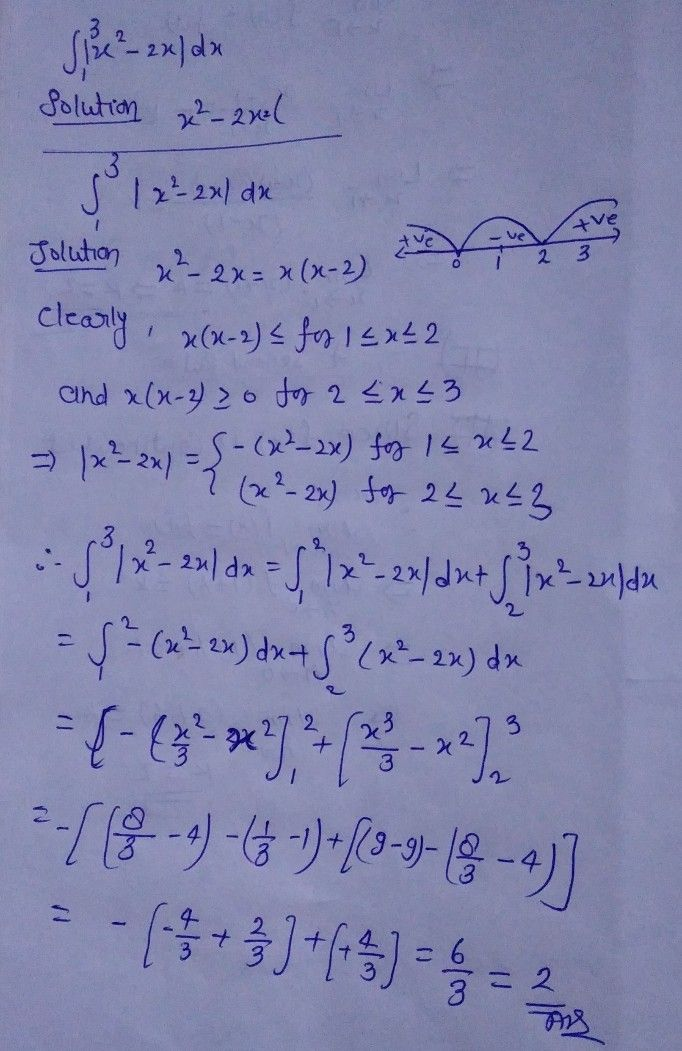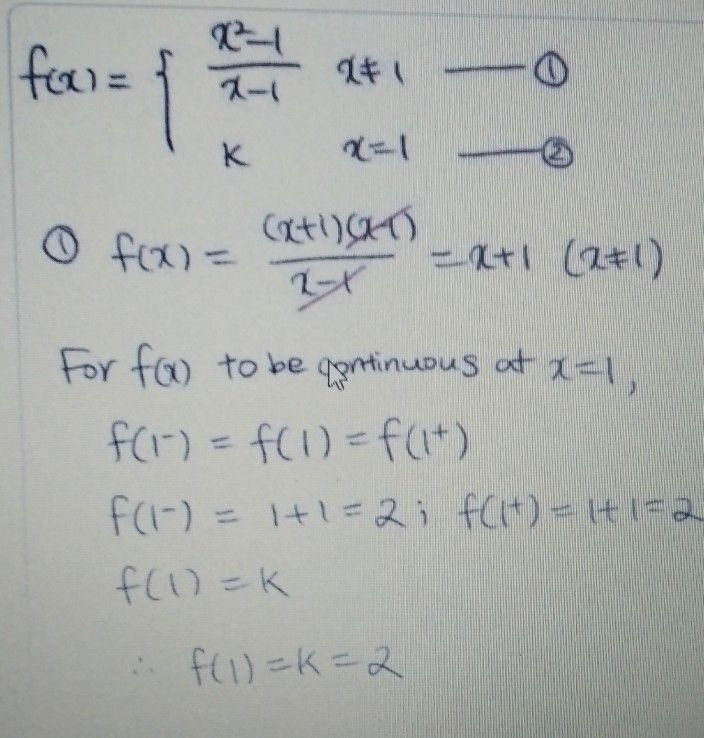Symbol
Problem$20$ Evaluate $\int ^{3}|x^{2}-2x|$ $dx$ $1$ $30$ when $x≠1$ If the function $f\left(x\right)=\left(\dfrac {x^{2}-1} {x-1}$ $k$ when $x=1$ is given to be continuous at $x=1,$ then the value of $k$ $1S$
Physics
Search count: 1,555
SolutionQanda teacher - BhanusirQanda teacher - ritumam